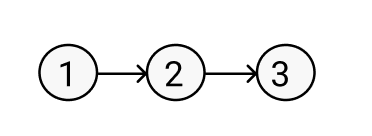New update is available. Click here to update.

# Ninja and his Courses

Last Updated: 25 Mar, 2021
Difficulty: Hard

## PROBLEM STATEMENT

#### For example:

``````Let‘N’ = 3 , ‘K’ = 1 and ‘DEPENDENCIES’ = [ [1, 2] [2, 3] ].
````````````In this example, if you want to take ‘COURSE_3’ then first you have to take ‘COURSE_2’ in the previous semester. If you want to take ‘COURSE_2’, then first you have to take ‘COURSE_1’ in the previous semester.
The value of ‘K’ is 1 which means in a semester we can choose at most 1 course.
``````
##### Input Format
``````The first line of input contains an integer ‘T’ which denotes the number of test cases or queries to be run. Then the test cases follow.

The first line of each test case contains three single space-separated integers ‘N’,‘M’ and ‘K’ represent the number of courses, number of dependencies and maximum courses that you can take in one semester respectively.

The next ‘N’ line of each test case contains two single space-separated integers ‘X’ and ‘Y’ representing that the course ‘X’ must be taken before the course ‘Y’.
``````
##### Output Format :
``````For each test case, print the minimum number of semesters required to take all the courses.

Print the output of each test case in a separate line.
``````

#### Note:

``````You do not need to print anything; it has already been taken care of. Just implement the given function.
``````
##### Constraints:
``````1 <= ‘T’ <= 10
1 <= ‘N’ <= 15
0 <= ‘M’ <= ‘N’ * (‘N’ - 1)/2
1 <= ‘X’, ‘Y’ and ‘K’ <= ‘N’

Time Limit: 1 second
``````## Approach 1

As we know we can only take a course which is not dependent on any other course. So first we make a graph having edges ‘DEPENDENCIES[i]’ and nodes represent each course. Then we find all the nodes whose in-degree is 0 i.e. these nodes don’t depend on any other nodes. If the nodes are less than ‘K’ then we take all the nodes and subtract the indegree of all the nodes which are connected through these nodes. Else we are trying to select ‘K’ nodes by all possible ways from the current set of nodes and find our answer.

Here is the algorithm:

1. We declare a ‘DP’ array/list where ‘DP[i]’ represents the minimum semesters required to take all the courses.
2. We declare a ‘GRAPH’ list/vector and we make this graph by ‘DEPENDENCIES’ array/list where ‘DEPENDENCIES[i]’  represents the edge of this ‘GRAPH’.
3. We declare a variable ‘MASK’ in which stores all the courses taken by us till now.
4. We call our helper function ‘minSemHepler(‘MASK’).
5. Finally, we return the answer which is calculated by our helper function.

1. Base case: If all courses are taken:
1. Return 0.
2. If ‘DP[‘MASK’]’ != -1 i.e if we previously calculated the result for these courses:
3. We declare an ‘INDEGREE’ list/vector in which we store that the particular course is dependent on how many other courses.
4. We run a loop for ‘i’ = 0 to ‘N’:
1. If the current course is already taken:
1. Continue.
2. We run a loop for ‘j’ = 0 to ‘GRAPH[i].size()’:
1. ‘INDEGREE[i] ++’.
5. We declare a variable ‘AVIL_MASK’ in which we store which courses are left behind that are not taken by you till now.
6. We run a loop for ‘i’ =  0 to ‘N’:
1. If ‘INDEGREE[i] is 0 and ‘i’th bit in ‘MASK’ is not set:
1. Set the ‘’’h bit in ‘AVIL_MASK’.